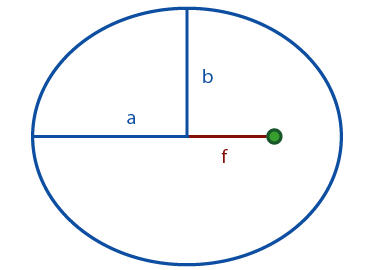Gets the eccentricity of the orbit.

Namespace:  AGI.Foundation.Coordinates
Assembly:  AGI.Foundation.OrbitPropagation (in AGI.Foundation.OrbitPropagation.dll) Version: 23.2.417.0 (23.2.417.0)Syntax
`public double Eccentricity { get; }`

#### Property Value

Type: DoubleRemarks

Eccentricity e can be calculated on the basis of semimajor axis a and semiminor axis b as follows:

`e = sqrt(1 - (b / a)^2)`

Referring to the following diagram, e can also be expressed as a / f, where f is the distance from the center of the ellipse to the center of mass of the central body:See Also